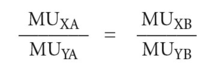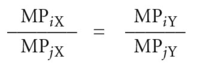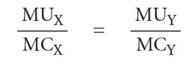# Pareto optimality

Also found in: Encyclopedia.

## Pareto optimality

the maximization of the economic welfare of the community.

There are three conditions that must hold in order for a Pareto optimum to be attained.

Consider a simplified economy in which there are two goods produced (X and Y) and two consumers (A and B).

1. optimal distribution of goods between consumers requires that:MUYB where MUXA is the MARGINAL UTILITY of good X to consumer A, MUYA is the marginal utility of good Y to consumer A, and so forth. This condition states that the MARGINAL RATE OF SUBSTITUTION between two goods (the ratio of their marginal utilities) must be the same for each consumer. If this were not the case, the consumers could improve their positions by exchanging goods. The consumer who values X highly relative to Y could trade some of his Y to the consumer who values Y highly relative to X. Only when the utility ratios are the same for both consumers is such mutually beneficial trade impossible.

INDIFFERENCE CURVES that show combinations of goods that yield satisfaction to consumers can be used to depict marginal rates of substitution insofar as their slopes reflect these trade-offs.

2. optimal allocation of inputs in productive uses where two inputs i and j are used in the production of goods X and Y. Optimal utilization of inputs requires that the ratio of the MARGINAL PHYSICAL PRODUCTS of i and j employed in the production of X be the same as the ratio of their marginal physical products in the production of Y. That is:If this equality is not satisfied, one input is relatively more efficient in the production of one output (and the other input is thus relatively less efficient in the production of the same output). It will thus benefit the community to divert more of the first input into its more efficient use (and to divert the other input from that use). This will permit the community to expand the production of one output at a constant level of resource use. Only when the marginal product ratios are the same can the community not gain by reallocating its inputs among competing uses. Production ISOQUANTS, which show combinations of inputs that produce a given output, can be used to depict MARGINAL RATES OF TECHNICAL SUBSTITUTION insofar as their slopes reflect these trade-offs.

3. optimal amounts of output (illustrated in Fig. 139, which depicts a transformation curve (PRODUCTION POSSIBILITY BOUNDARY) shows the quantities of goods X and Y that can be produced by utilizing fully the resources of the community). The slope of this transformation curve represents the MARGINAL RATE OF TRANSFORMATION, that is, the ratio of the MARGINAL COST of good X to the marginal cost of good Y. Optimal output for any pair of goods, X and Y, requires that the goods be produced in quantities such that:that is, the ratio of marginal cost to marginal utility must be the same for each good, so that the last £'s worth of good X generates the same utility as the last £'s worth of good Y, etc. This condition will be satisfied if the slope of the transformation curve (the marginal cost ratio between X and Y) is equal to the marginal utility ratio between X and Y (the marginal rate of substitution). If the ratios were unequal, the community would benefit by producing more of the good that yields the higher marginal utility per unit of marginal cost. The optimal combination of goods is depicted as OX1 ant OY1 in Fig. 139, where the indifference curve (IC) is tangential to the production possibility boundary (PP). It should be noted, however, that Pareto optimality indicates only the optimum allocation of resources for a given pattern of demand, which itself reflects the present PERSONAL. DISTRIBUTION OF INCOME. Any change in the distribution of personal income, say from rich to poor (see REDISTRIBUTION OF INCOME), would change demand patterns for products and lead to a different Pareto optimum.

If all these conditions are met, then it would be impossible to improve the welfare of one or more individuals without simultaneously reducing the welfare of another or others. See EDGEWORTH BOX, WELFARE ECONOMICS.

Collins Dictionary of Economics, 4th ed. © C. Pass, B. Lowes, L. Davies 2005
References in periodicals archive ?
In this work, a novel approach to the design of the HTS cable cross-section, based on the Pareto optimality criterion, is proposed that may represent a useful tool for the designer to choose among different cable's configurations for electric power transmission, depending on the relative "importance" given to the current density [J.sub.eng] with respect to the total current [I.sub.C.sup.cable].
However, there is a drawback in finding true Pareto optimality while the proposed method is more capable of finding more optimality points in better quality and discipline.
To illustrate the Pareto optimality mathematically, consider two design vectors u, v [member of] S; then vector u is said to be dominant over vector v (denoted by u < v) iff [for all]I [member of] {1, 2, ..., m}, [F.sub.l](u) [less than or equal to] [F.sub.l] (v) ^ [there exists]1 [member of] {1, 2, ..., m} [F.sub.l](u) < Fi(v).
In terms of our GWAS datasets research article, on the basis of unified framework which contains fast adaptive ant colony optimization (ACO) algorithm, Akaike Information Criterion (AIC) score, explain score, and Pareto optimality, we can obtain the final result which is a nondominated SNP set; in this section, we will use Fisher exact test to exhaustively search for the epistasis effect.
Pareto optimality might usefully be said to be what Kant called a 'regulative' idea or principle.
Generally, the term "efficiency" refers to the concept of Pareto optimality. The Pareto principle embodies the idea that actual consensus allows social decisionmaking to achieve ethical neutrality.
(8) We have also derived the necessary first-order condition for first best Pareto optimality. For the theorem to hold for the insurer's utility level derived from its endowment, [[gamma].sub.IN] = [c.sub.0], we have to check case by case whether [c.sub.0] [member of] [[gamma].sub.IN].
As the obtained design values are evaluated against multiple criteria, Pareto optimality concept is applied in the analysis phase.
All players' strategies should be in favor of achieving Pareto optimality. Obviously, in the above game model, the expected equilibrium is the decentralization, work hard strategy, aimed at promoting academic freedom and academic development.
(30) "[Jjustice is a multi-attributed quality" and "Pareto optimality is a relatively minor attribute of that quality." (31) As Sager further explained, the Pareto principle cannot reliably track the requirements of justice because it focuses on pair-wise comparisons: How does the status quo compare to a proposed alternative state of affairs?

Site: Follow: Share:
Open / Close